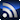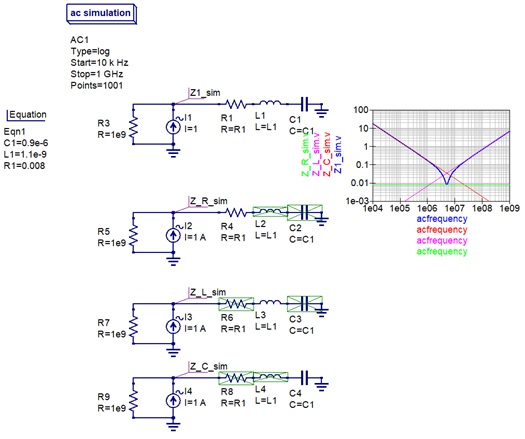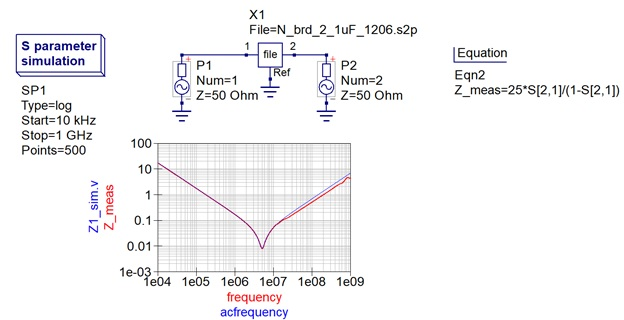# Eric Bogatin, Signal Integrity Journal Technical EditorRSSEric Bogatin is Technical Editor at Signal Integrity Journal and the Dean of the Teledyne LeCroy Signal Integrity Academy. Additionally, he is an Adjunct Professor at the University of Colorado - Boulder in the ECEE Dept. Eric improves the signal to noise ratio by sorting through all of the information available and finding the best quality content to publish on signalintegrityjournal.com.

Signal Integrity

# A Free Impedance Analyzer on your Desktop

December 16, 2021

Impedance is the most fundamental and important electrical property of any component. With a very simple technique, anyone can simulate the impedance of any circuit in seconds using any version of SPICE, for free.

SPICE is a tool that will simulate the voltage or current on any node of any circuit in the time domain or the frequency domain. SPICE is to an electrical engineer like a calculator is to an accountant: an essential tool you will use multiple times every day. It should be effortless to use so there are no barriers to using it when needed. While there are many versions around, two stand out as particularly easy to use and free: CircuitLab, an online version and QUCS, a downloadable version.

Whichever version you use, they all can simulate the impedance of any component. If you can draw the circuit with ideal circuit elements, you can simulate the impedance with a very simple circuit.

The definition of impedance, Z, in the frequency domain or the time domain, is Z = V/I. In the frequency domain, we can create a sine wave voltage source, apply it to a circuit and calculate the current through the circuit. The ratio is the impedance. But there is an even simple way of doing this using a constant current sine wave source.

Every version of SPICE has a built-in circuit element that is a constant current AC source. This means that no matter the load attached to this source, it will generate whatever voltage it needs in order to create a constant amplitude sine wave of current. If we set the amplitude to be 1 A amplitude, then the voltage across the circuit is just V = Z x I. If I = 1 Amp, then the voltage is just Z. Change the name of the units from volts to ohms and you have the impedance. Not only that, but the phase of the calculated voltage is the phase of the impedance.

As a starting place, always plot the simulated impedance on a log-log scale with frequency. This way the impedance of a resistor is a flat line, the impedance of a capacitor is a line with a slope of -1 and an inductor’s impedance is a line with a slope of +1. This is shown in Figure 1, simulated with QUCS.Figure 1. The impedance analyzer circuit set up in QUCS for an R, L and C circuit and individual elements.

This circuit illustrates three other features in QUCS: the ability to parameterize all values, being able to turn off elements in a circuit by either shorting them or opening them, and an integrated plotting tool that can be placed on any schematic page.

The 1e9 ohm resistor in parallel to every constant current AC source is there to guarantee a finite voltage in every situation no matter the circuit.

With this circuit, we can simulate the impedance of any circuit, however complex. Change a parameter value and the resulting impedance can be simulated and displayed on a log-log scale.

But that’s not all. If you also have the measured 2-port S-parameters of a component, like a capacitor, you can compare the measured impedance with the simulated impedance of an RLC circuit model. An example of measuring the impedance of real capacitors can be found in this article. Figure 2 shows the measured impedance of a 1 uF 1206 MLCC capacitor compared with the simulated impedance of a simple RLC circuit. The values of the R, L and C were adjusted (hacked) to match the measured impedance of the real capacitor.Figure 2. Comparing the measured and simulated impedance of a real MLCC capacitor.

After the five-minute learning curve to build circuits in QUCS and set up a simulation, you can build an impedance analyzer, bring in measured data and do your own hacking in a matter of minutes. It doesn’t get any easier than this. And it’s free.

When you graduate from QUCS and want a more powerful version of SPICE, I recommend Keysight’s ADS. While it is very powerful, it is not free. Maybe someday, they will offer a free student version.